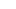# Valentines Day Math – Prime Numbers for Kids Sorting Activity

If you are looking for a fun valentine’s day math activities, you will love this valentines day math that helps kids practice sorting prime and composite numbers. In this prime numbers activity, grade 3 and grade 4 students will use the free printable heart numbers and sorting mats to practice sorting numbers.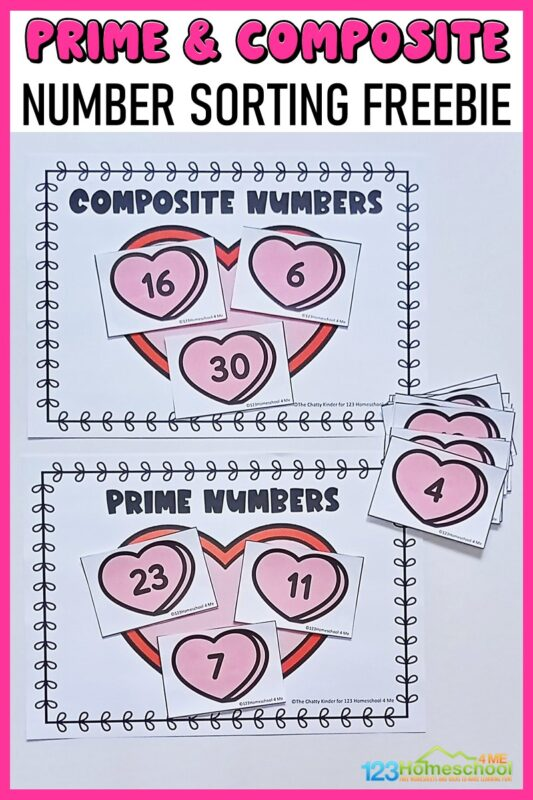## Valentines Day Math

Looking for a fun math activity for your 3rd and 4th graders? This prime and composite number sorting activity is perfect to practice those tricky math skills. The printable is valentine themed, making it perfect for a valentine themed unit activity. Practice the concept of factors and multiplication with your 3rd and 4th graders using this fun and hands-on printable. Teaching multiplication is tricky and can be confusing so make learning it fun with this hands-on sorting activity printable.

## Prime numbers for kids

Start by scrolling to the bottom of the post, under the terms of use, and click on the text link that says >> _____ <<. The pdf file will open in a new window for you to save the freebie and print the template.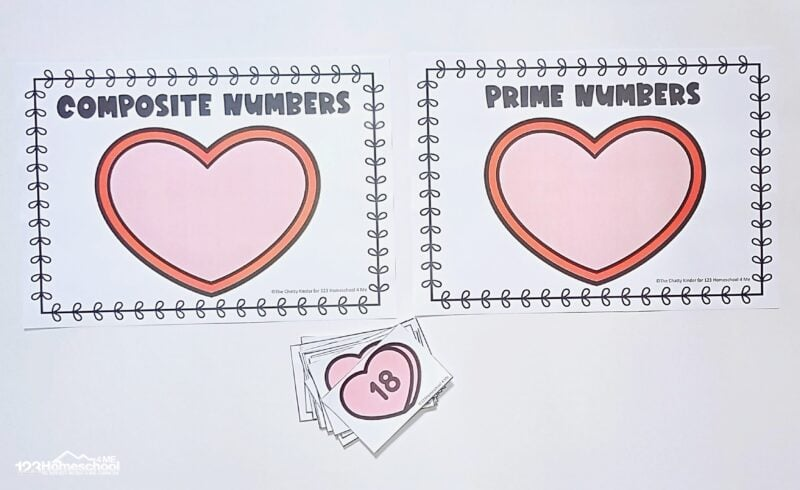## What are Factors?

When learners first begin learning multiplication and division, they start understanding the concept of factors. A factor is the number which is multiplied by another whole number to give a product. For e.g. 4×3 gives 12, and in this case 3 and 4 are the factors of 12. Once learners are familiar with the concept of factors they can then be introduced to the concept of prime and composite numbers.

## Prime Numbers and Composite numbers

A prime number is a number greater than 1 which only has 2 factors, 1 and itself. That means it is not divisible by any other number apart from these two numbers. Some examples include 2, 3, 5, 7, 11, etc.

A composite number is a positive integer that can be formed by multiplying two smaller positive integers. It has at least 3 factors (including one and the number itself). Examples of composite numbers included 4, 6, 8, 9, 10, etc.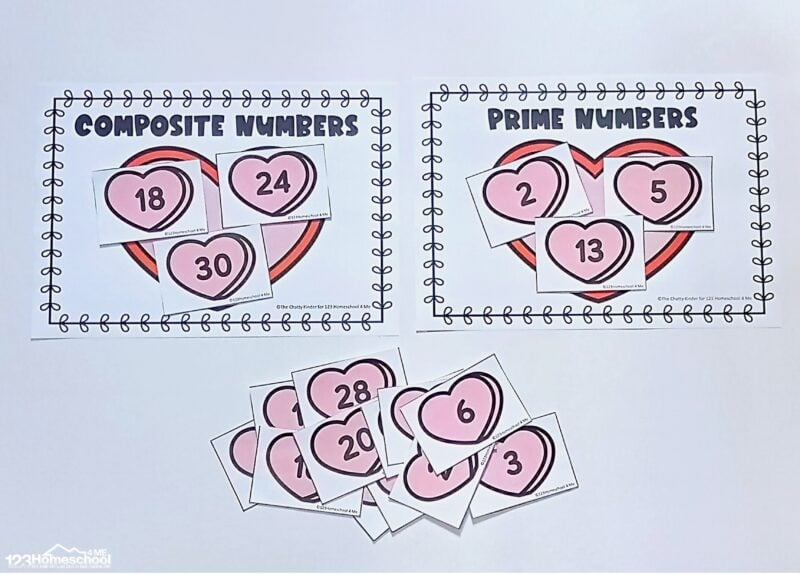## Why are prime and composite numbers important?

Math is built on numbers and understanding numbers is the very foundation of math. When factorizing numbers it can be seen that even the composite numbers in the number can be further factorized into prime numbers. This makes prime numbers the basic building blocks of numbers. Numbers can be formed by endlessly multiplying prime numbers over and over again. This helps to further strengthen understanding of the number sense in numbers and can also help to solve puzzles and problems in maths.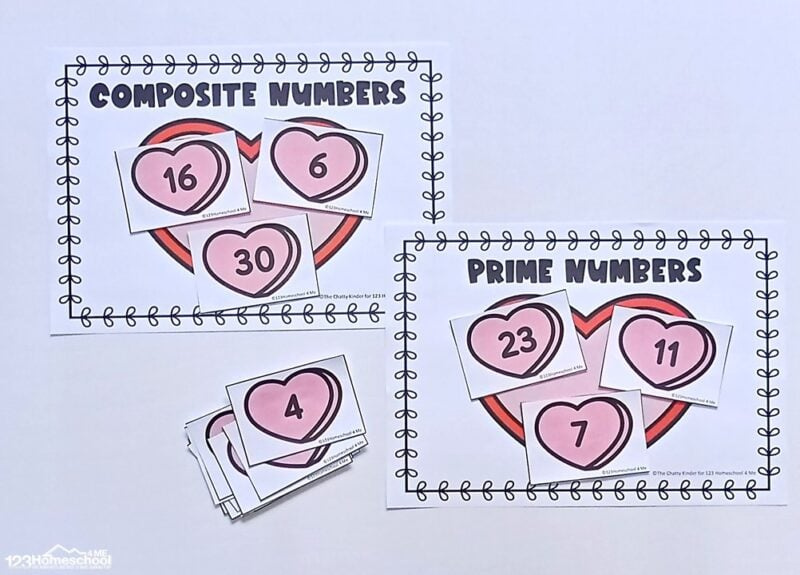## Sorting Prime and Composite Numbers Printable

This printable uses hearts for sorting which makes this activity perfect for a Valentine’s Day math activity. Prepping this printable is very simple.

• Simply print out the pages and laminate if desired. Cut apart the pages to create the sorting number cards.
• Present the learners with the two sorting mats.

Learners will pick up each number card and determine if the number is prime or composite based on their understanding of the factors. This should be explained to the students prior to starting on the sorting activity. Learners will place the numbers onto the correct sorting mats.

The printable includes all the prime numbers from 1-100 and some of the composite numbers within 1-100. If the learner is just starting to get introduced to the concept of prime and composite numbers start off with a few numbers, since it can tire out the learner easily.

Learners can also record down the numbers within each category once they are done sorting if they wish to record their work.

## February Math

By using resources from my site you agree to the following:

• This is for personal use only (teachers please see my TPT store)
• This may NOT be sold, hosted, reproduced, or stored on any other site (including blog, Facebook, Dropbox, etc.)